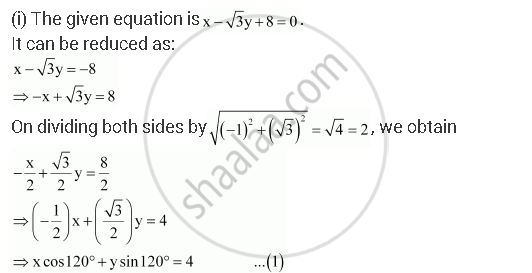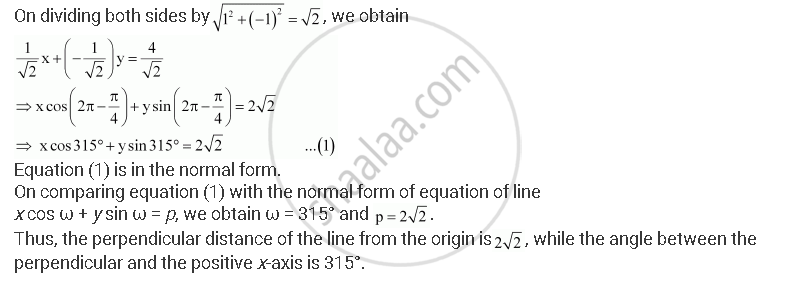CBSE (Arts) Class 11CBSE
Share

# Reduce the Following Equations into Normal Form. Find Their Perpendicular Distances from the Origin and Angle Between Perpendicular and the Positive X-axis. - CBSE (Arts) Class 11 - Mathematics

ConceptGeneral Equation of a Line

#### Question

Reduce the following equations into normal form. Find their perpendicular distances from the origin and angle between perpendicular and the positive x-axis.

i) x – sqrt3y + 8 = 0

(ii) – 2 = 0

(iii) – = 4

#### SolutionEquation (1) is in the normal form.

On comparing equation (1) with the normal form of equation of line

x cos ω + y sin ω = p, we obtain ω = 120° and p = 4.

Thus, the perpendicular distance of the line from the origin is 4, while the angle between the perpendicular and the positive x-axis is 120°.

(ii) The given equation is – 2 = 0.

It can be reduced as 0.x + 1.= 2

On dividing both sides bysqrt(0^2 + 1^2) = 1, we obtain 0.x + 1.= 2

⇒ x cos 90° + sin 90° = 2 … (1)

Equation (1) is in the normal form.

On comparing equation (1) with the normal form of equation of line

x cos ω + y sin ω = p, we obtain ω = 90° and p = 2.

Thus, the perpendicular distance of the line from the origin is 2, while the angle between the perpendicular and the positive x-axis is 90°.

(iii) The given equation is – = 4.

It can be reduced as 1.x + (–1) = 4Is there an error in this question or solution?

#### Video TutorialsVIEW ALL 

Solution Reduce the Following Equations into Normal Form. Find Their Perpendicular Distances from the Origin and Angle Between Perpendicular and the Positive X-axis. Concept: General Equation of a Line.
S